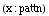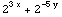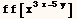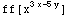Pattern  (x:pattn)

Mathematica lets us use (x_Foo) to say that something with the head Foo will be  called (x).  It also lets us use (x_?Test)  to say something for which Test  returns True will be called (x).  Now if we want to say that something  matching a certain pattern is called (x) we use.

The next cell gives an example where use of Pattern is helpful.  Here (ff) is
only defined when it's argument is an Integer raised to a power.  In addition
the exponent must have the head Plus.In the example below we get the result of evaluating.Next we see (ff) is undefined when it's argument isn't an Integer raised to a
power where the exponent has the head Plus.Created by Mathematica  (May 16, 2004)

Back to Ted’s Tricks index page